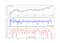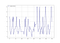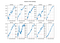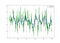# Creating a Variable RSI for Dynamic Trading. A Study in Python.

## How to Create a Dynamic RSI to Enhance Trading Signals.

In the last article, we have seen together how to create a Volatility-Adjusted Stochastic Oscillator. In this article, we will use the same reasoning on the RSI but we will adjust for correlation rather than volatility. The basic idea is to see whether we want to weigh the RSI’s lookback period based on a rolling correlation measure.

Our plan of attack will be to learn how to code an RSI that changes its lookback period at every period in time based on the latest rolling correlation measure between the price and a momentum calculation. The reason I have chosen a momentum calculation is that because we do not know the lookback period on the RSI yet and therefore, we cannot really calculate a correlation measure on it specifically. When we know the correlation between the momentum and the market price, we can transform this information into variable lookback periods for the RSI. We will see this in greater details below. If you are interested in the previous article where we adjusted the Stochastic Oscillator for volatility and back-tested a trading strategy, you can check out the below link:

# The Relative Strength Index

We all know about the Relative Strength Index — RSI and how to use it. It is without a doubt the most famous momentum indicator out there, and this is to be expected as it has many strengths especially in ranging markets. It is also bounded between 0 and 100 which makes it easier to interpret. Also, the fact that it is famous, contributes to its potential.

This is because, the more traders and portfolio managers look at the RSI, the more people will react based on its signals and this in turn can push market prices. Of course, we cannot prove this idea, but it is intuitive as one of the basis of Technical Analysis is that it is self-fulfilling.

J. Welles Wilder came up with this indicator in 1978 as a momentum proxy with an optimal lookback period of 14 periods. It is bounded between 0 and 100 with 30 and 70 as the agreed-upon oversold and overbought zones respectively. The RSI can be used through 4 known techniques:

• Oversold/Overbought zones as indicators of short-term corrections.
• Divergence from prices as an indication of trend exhaustion.
• Drawing graphical lines on the indicator to find reaction levels.
• Crossing the 50 neutrality level as a sign of a changing momentum.

The RSI is calculated using a rather simple way. We first start by taking price differences of one period. This means that we have to subtract every closing price from the one before it. Then, we will calculate the smoothed average of the positive differences and divide it by the smoothed average of the negative differences. The last calculation gives us the Relative Strength which is then used in the RSI formula to be transformed into a measure between 0 and 100.

The smoothed version used in J. Welles Wilder’s calculations is related to the common exponential average that we are used to.

The below code snippet shows how to calculate an exponential moving average and the RSI. I have provided the first function because it is used in the RSI formula in the form of a smoothed moving average. Here is an example:

`def ema(Data, alpha, lookback, what, where):        # alpha is the smoothing factor    # window is the lookback period    # what is the column that needs to have its average calculated    # where is where to put the exponential moving average        alpha = alpha / (lookback + 1.0)    beta  = 1 - alpha        # First value is a simple SMA    Data = ma(Data, lookback, what, where)        # Calculating first EMA    Data[lookback + 1, where] = (Data[lookback + 1, what] * alpha) + (Data[lookback, where] * beta)# Calculating the rest of EMA    for i in range(lookback + 2, len(Data)):            try:                Data[i, where] = (Data[i, what] * alpha) + (Data[i - 1, where] * beta)                    except IndexError:                pass    return Datadef rsi(Data, rsi_lookback, what1, what2):        rsi_lookback = (rsi_lookback * 2) - 1 # From exponential to smoothed              # Get the difference in price from previous step    delta = []       for i in range(len(Data)):        try:            diff = Data[i, what1] - Data[i - 1, what1]             delta = np.append(delta, diff)                          except IndexError:            pass            delta = np.insert(delta, 0, 0, axis = 0)                   delta = delta[1:]         # Make the positive gains (up) and negative gains (down) Series    up, down = delta.copy(), delta.copy()    up[up < 0] = 0    down[down > 0] = 0        up = np.array(up)    down = np.array(down)        roll_up = up    roll_down = down        roll_up = np.reshape(roll_up, (-1, 1))    roll_down = np.reshape(roll_down, (-1, 1))        roll_up = adder(roll_up, 3)    roll_down = adder(roll_down, 3)        roll_up = ema(roll_up, 2, rsi_lookback, what2, 1)    roll_down = ema(abs(roll_down), 2, rsi_lookback, what2, 1)        # Calculate the SMA    roll_up = roll_up[rsi_lookback:, 1:2]    roll_down = roll_down[rsi_lookback:, 1:2]    Data = Data[rsi_lookback + 1:,]        # Calculate the RSI based on SMA    RS = roll_up / roll_down    RSI = (100.0 - (100.0 / (1.0 + RS)))    RSI = np.array(RSI)    RSI = np.reshape(RSI, (-1, 1))    RSI = RSI[1:,]        Data = np.concatenate((Data, RSI), axis = 1)return Data`

A smoothed moving average of 200 periods is the same as an exponential moving average of 399. This means that to transform from smoothed to exponential, we can multiply by two and subtract one.

However, in this article, we are not interested in the regular RSI. We will instead, calculate the rolling 20-period correlation between a momentum measure and the currency pair and then determine for each period, the optimal lookback period for the RSI before calculating the Dynamic RSI.

Hence, our variable (unknown element) in our equation will be the lookback period. We will try an interesting way of finding it based on the strength of the Momentum correlation.

So, the quest becomes to find a correlation-weighted RSI based on the price’s momentum. The description is more complicated than the reality. Let us start building block by block.

# Creating the Dynamic RSI

The first step is to calculate the momentum of the price based on the last 5 periods. This is done by dividing the last closing price by the closing price 5 periods ago:

We can code it through this function:

`def momentum_indicator(Data, what, where, lookback):     for i in range(len(Data)):     Data[i, where] = Data[i, what] / Data[i - lookback, what] * 100        return Data# The Data variable is the OHLC array# The what variable is the closing price column# The where variable is where to put the indicator# The lookback variable is the subtraction range which is 5`

The next step is to calculate a 20-period rolling correlation measure between the price and the momentum calculation.

Correlation is the degree of linear relationship between two or more variables. It is bounded between -1 and 1 with one being a perfectly positive correlation, -1 being a perfectly negative correlation, and 0 as an indication of no linear relationship between the variables (they relatively go in random directions). The measure is not perfect and can be biased by outliers and non-linear relationships, it does however provide quick glances to statistical properties. The most common correlation measure is called the Pearson’s correlation and it is the one we will be using for the remainder of the article.

`from scipy.stats import pearsonrdef rolling_correlation(Data, first_data, second_data, lookback, where):        for i in range(len(Data)):                try:            Data[i, where] = pearsonr(Data[i - lookback + 1:i + 1, first_data], Data[i - lookback + 1:i + 1, second_data])                                except ValueError:            pass        Data = jump(Data, lookback)         return Data`EURUSD in the first panel, 5-period momentum in the second panel, and their 20-period rolling correlaiton. (Image by Author)

The last step before creating the dynamic RSI is to transform the correlation measure into variable lookback periods at each step in time. This means that we will be replacing the correlation values with integers based on the conditions below:

• Whenever the corelation between price and its 5-period momentum is relatively high according to the latest 20 periods, we should bias the RSI lookback period downwards to account more for the recent values.
• Whenever the corelation between price and its 5-period momentum is relatively low according to the latest 20 periods, we should bias the RSI lookback period upwards to account less for the recent values.

We can use more complex stuff to account for the lookbacks but we can try something extremely simple. Here is how:

• Whenever the correlation is between -1.00 and 0.10, the lookback period of the RSI is 14.
• Whenever the correlation is between 0.10 and 0.20, the lookback period of the RSI is 10.
• Whenever the correlation is between 0.20 and 0.30, the lookback period of the RSI is 9.
• Whenever the correlation is between 0.30 and 0.40, the lookback period of the RSI is 8.
• Whenever the correlation is between 0.40 and 0.50, the lookback period of the RSI is 7.
• Whenever the correlation is between 0.50 and 0.60, the lookback period of the RSI is 6.
• Whenever the correlation is between 0.60 and 0.70, the lookback period of the RSI is 5.
• Whenever the correlation is between 0.70 and 0.80, the lookback period of the RSI is 4.
• Whenever the correlation is between 0.80 and 0.90, the lookback period of the RSI is 3.
• Whenever the correlation is between 0.90 and 1.00, the lookback period of the RSI is 2.A sample of how the lookback on the RSI changes with the change in correlation. (Image by Author)

This is a way to gradually weigh the RSI lookback periods. Note that you can select whichever periods you want and optimize them according to your preferences. The default parameters on the Dynamic RSI (according to me) are the ones above.

Let us now see the full function that gives out this indicator before we proceed to the back-testing step. Note that you must use it on an OHLC array with multiple extra columns to be populated by the function.

`def dynamic_rsi(Data, momentum_lookback, corr_lookback, what, where):            for i in range(len(Data)):        Data[i, where] = Data[i, what] / Data[i - momentum_lookback, what] * 100Data = rolling_correlation(Data, what, where, corr_lookback, where + 1)        for i in range(len(Data)):                if Data[i, where + 1]>= -1.00 and Data[i, where + 1]<= 0.10:            Data[i, where + 1] = 14        if Data[i, where + 1] > 0.10 and Data[i, where + 1]<= 0.20:            Data[i, where + 1] = 10                    if Data[i, where + 1] > 0.20 and Data[i, where + 1]<= 0.30:            Data[i, where + 1] = 9        if Data[i, where + 1] > 0.30 and Data[i, where + 1]<= 0.40:            Data[i, where + 1] = 8        if Data[i, where + 1] > 0.40 and Data[i, where + 1]<= 0.50:            Data[i, where + 1] = 7        if Data[i, where + 1] > 0.50 and Data[i, where + 1]<= 0.60:            Data[i, where + 1] = 6        if Data[i, where + 1] > 0.60 and Data[i, where + 1]<= 0.70:            Data[i, where + 1] = 5        if Data[i, where + 1] > 0.70 and Data[i, where + 1]<= 0.80:            Data[i, where + 1] = 4        if Data[i, where + 1] > 0.80 and Data[i, where + 1]<= 0.90:            Data[i, where + 1] = 3        if Data[i, where + 1] > 0.90 and Data[i, where + 1]<= 1.00:            Data[i, where + 1] = 2                    Data = rsi(Data, 14, 3, 0)    Data = rsi(Data, 10, 3, 0)    Data = rsi(Data, 9, 3, 0)    Data = rsi(Data, 8, 3, 0)    Data = rsi(Data, 7, 3, 0)    Data = rsi(Data, 6, 3, 0)    Data = rsi(Data, 5, 3, 0)    Data = rsi(Data, 4, 3, 0)    Data = rsi(Data, 3, 3, 0)    Data = rsi(Data, 2, 3, 0)for i in range(len(Data)):                if Data[i, where + 1] == 14:            Data[i, where + 12] = Data[i, where + 2]        if Data[i, where + 1] == 10:            Data[i, where + 12] = Data[i, where + 3]        if Data[i, where + 1] == 9:            Data[i, where + 12] = Data[i, where + 4]        if Data[i, where + 1] == 8:            Data[i, where + 12] = Data[i, where + 5]        if Data[i, where + 1] == 7:            Data[i, where + 12] = Data[i, where + 6]        if Data[i, where + 1] == 6:            Data[i, where + 12] = Data[i, where + 7]        if Data[i, where + 1] == 5:            Data[i, where + 12] = Data[i, where + 8]        if Data[i, where + 1] == 4:            Data[i, where + 12] = Data[i, where + 9]        if Data[i, where + 1] == 3:            Data[i, where + 12] = Data[i, where + 10]        if Data[i, where + 1] == 2:                        Data[i, where + 12] = Data[i, where + 11]return Data`

Now, how do the results of the Dynamic RSI compare to the results of the regular RSI? After all, we need a benchmark or some form of comparison to properly judge our strategy. I will make an exception in this back-test regarding the risk management processes I employ, as I will rather respect the optimal risk-reward ratio of 2.00. This means that I will place my stops at 1x the 50-period ATR and my targets at the 2x 50-period ATR. Another way of saying that I will be risking half of what I expect in each trade.

The below graphs show the performance tables and the equity curves of a strategy that:

• Goes long (Buy) whenever the indicator reaches 30 and hold the position until a new signal is generated or until the position is stopped-out either by a stop-loss or a profit order.
• Goes short (Sell) whenever the indicator reaches 70 and hold the position until a new signal is generated or until the position is stopped-out either by a stop-loss or a profit order.Equity Curves following the RSI strategy on the left, and following the Dynamic RSI strategy on the right. (Image by Author)

What is impressive with the Dynamic RSI is the fact that it adds value considering a 2.00 risk reward ratio. This has the potential to reduce risk and augment returns. For more about correlation in case you need a refresher, you can check out this article:

While it is better to use the Dynamic RSI (Also known as the Correlation-weighted RSI) in systematic strategies because we can get a glance at its efficacy in a matter of minutes, it is also possible to use it in discretionary trading through the same old graphical techniques described above.

It becomes interesting to use the Dynamic RSI even though the regular RSI is more closely watched by traders out there. The difference between the two does seem to show that we have a another indicator on our hands. Maybe one day we can combine both signals?The 14-period RSI and the Dynamic RSI in one plot. (Image by Author)

# Conclusion

Why was this article written? It is certainly not a spoon-feeding method or the way to a profitable strategy. If you follow my articles, you will notice that I place more emphasize on how to do it instead of here it is and that I also provide functions not full replicable code. In the financial industry, you should combine the pieces yourself from other exogenous information and data, only then, will you master the art of research and trading.

I always advise you to do the proper back-tests and understand any risks relating to trading. For example, the above results are not indicative as the spread we have used is very competitive and may be considered hard to constantly obtain in the retail trading world (but not impossible). However, with institutional bid/ask spreads, it may be possible to lower the costs such as that a systematic medium-frequency strategy starts being very profitable .You must never expect guaranteed returns and it is your sole responsibility to generate your own signals.

Written by

Written by

## Sofien Kaabar

#### Institutional FOREX Strategist | Trader | Data Science Enthusiast. Author of the Book of Back-tests: https://www.amazon.com/dp/B089CWQWF8